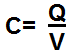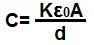﻿ Capacitance Calculator ﻿# Capacitance Calculator### Capacitance Calculator If Charge and Voltage Are KnownpC nC µC mC C

V

0.0000000000F

This capacitance calculator calculates the capacitance of a capacitor based on its charge, Q, and its voltage, V, according to the formula, C=Q/V.

A user enters the charge, Q, and the voltage, V and the result will automatically be calculated and shown. The capacitance result which is displayed above is in unit farads (F).

Measuring a capacitor's capacitance is very important for many electronic circuits because the capacitance value shows how much charge a capacitor can hold at a given voltage. For many circuits, capacitance is key because many circuits function on the amount of charge a capacitor can store to later dump out into the circuit. One such circuit that functions on this principle is a flash camera, one of those cameras where you wind up the camera to charge it and then later to press the switch to flash a picture. When you are winding this switch, you are charging the capacitor. The capacitance of the capacitor determines how much charge it can store at a certain voltage and measures its viability for a circuit such as this one.

### Capacitance Calculator If Area, Distance, and Dielectric Constant Are KnownDielectric Constant (K): Area (A): m2 Distance (D): m

nanF

This parallel plate capacitance calculator calculates the capacitance of a capacitor based on the area of the plate of the capacitor, the distance between the 2 plates of the capacitor, and the dielectric constant of the material which is present between the capacitor.

A user enters the dielectric constant, K, the area, A, and the distance, D, and the result will automatically be calculated and shown. The capacitance result which is displayed above is in unit farads (F).

The capacitance value increases with a larger area and decreases with a smaller area. This is because the more area a capacitor has, the more room or space there are for charges to rest on the plate surface. The capacitance increases when the distance between the plates comes closer together (is smaller) and decreases when the distance gets farther away (is larger). This is because closer distance results in greater electric field force. The last factor affecting capacitance is the dielectric constant of the material in between the plates of the capacitor. Some common dielectrics include a vacuum, air, and a range of other materials including polystyrene, polycarbonate, aluminum oxide, and tantalum pentoxide.

Below is a list of some common dielectrics used for capacitors with their corresponding dielectric constant values.

Dielectric Constants
 Vacuum 1 Air 1.00054 Polystyrene 2.4-2.7 Polycarbonate 3.1 Paper 3.5 Ruby mica 6.5-8.7 Aluminum oxide 8.4 Tantalum pentoxide 28

Related Resources

﻿